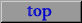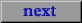## Intuiting Mathematical Objects Using Diagrams and Kinetigrams *

### John Pais(pais@kinetigram.com)

#### St. Louis College of Pharmacy

1. Philosophical Background: Doing Mathematics

Teachers of mathematics first and foremost want their students to learn how to do mathematics. However, there is no unanimity on answers to the questions: "What is mathematics?" and "How do you do it?" Perhaps too much emphasis has been placed on trying to answer the first, and not enough on addressing the second independently of an achieved consensus on the first. In   pages 148-150, Quine comments on Russell's witty, though extreme, answer to the first question:

 There has been a perverse tendency to think of mathematics primarily as abstract or uninterpreted and only secondarily as interpreted or applied, and then to philosophize about application. This was the attitude of Russell at the turn of the century, when he wrote that in pure mathematics "we never know what we are talking about, nor whether what we are saying is true." …This disinterpretation of mathematics was a response to non-Euclidean geometry. Geometries came to be seen as a family of uninterpreted systems… I find this attitude perverse [both in geometry and in arithmetic]. The words 'five' and 'twelve' are at no point uninterpreted; they are as integral to our interpreted language as the word 'apple' itself. They name two intangible objects, numbers, which are sizes of sets of apples and the like…  The expressions 'five', 'twelve', and 'five plus twelve' differ from 'apple' in not denoting bodies, but this is no cause for disinterpretation; the same can be said of such unmathematical terms as 'nation' or 'species'. Ordinary interpreted scientific discourse is as irredeemably committed to abstract objects--to nations, species, numbers, functions, sets--as it is to apples and other bodies.

Mathematical objects are abstract, in that we can posit them and reason about their properties and derivative objects, unencumbered by the necessity of their having any physical attributes. Generally, as indicated by Quine, a mathematical theory has an intended interpretation that guides the development of the theory and, as such, is intrinsic to an understanding of the theory and its intended mathematical objects. With this in mind, doing mathematics is not just an analytic process of proving theorems but an interplay between the analytic process and an intuitive unfolding of the mathematical objects one is trying to develop. So, doing mathematics is an heuristic process and it is this notion of 'doing' that we should try to communicate to our students. First and foremost, in order for mathematics to make sense, students need to learn how to intuit mathematical objects on their own.

I will now briefly discuss some recent related work. The best contemporary account of a realist philosophy of mathematics, in terms of clarity, coherence, and completeness, is Resnik's book  . In particular, he presents a lucid account of mathematical objects as posits. Though he ultimately argues for mathematical objects as positions in patterns, his primary discussion of mathematical objects does not depend on this interpretation. The following is from his introduction to Chapter 9. Positing Mathematical Objects, pages 175-176:

 …[I]n so far as realists maintain that mathematical objects are causally inert and outside space-time, they should explain how we can attain mathematical knowledge using just our ordinary faculties. I will now attempt to meet this challenge through a postulational account of the genesis of our mathematical knowledge. The basic idea is that humans brought mathematical objects into their ken by positing them. Now to posit a new kind of object one need only introduce a new predicate P (or, as happens frequently, begin to use an old one with a new sense) and claim that P exists. Thus, it is plain that realists who claim that mathematical objects are posits invite a variety of worries and objections. Postulational approaches seem better suited to conventionalists, who may claim that we make truths, than to realists, who must hold that we can only recognize independently obtaining truths. Below I will explain why positing is not incompatible with realism, I will distinguish positing in mathematics from the creative efforts of fiction writers, and, finally, I will explain how in positing mathematical objects we manage to refer to them. I will be assuming that in providing an epistemology for mathematics, realists are entitled to assume that we already have an abundant fund of knowledge of mathematical objects… The problem, then, is to explain how we have obtained the knowledge of mathematical objects we now have.

Hersh's book   does not seriously address traditional philosophy of mathematics, but many of his observations on doing mathematics provide valuable insights for teachers of mathematics. Specifically, some of his observations concerning mathematical intuition are quite interesting and helpful, e.g. pages 65-66:

 Accounting for intuitive "knowledge" in mathematics is the basic problem of mathematical epistemology. What do we believe, and why do we believe it? To answer this question we ask another question: what do we teach, and how do we teach it? Or what do we try to teach, and how do we find it necessary to teach it? We try to teach mathematical concepts, not formally (memorizing definitions) but intuitively--by examples, problems, developing an ability to think, which is the expression of having successfully internalized something. What? An intuitive mathematical idea… We have intuition because we have mental representations of mathematical objects. We acquire these representations, not mainly by memorizing formulas, but by repeated experiences (on the elementary level, experience of manipulating physical objects; on the advanced level, experiences of doing problems and discovering things for ourselves)… Different people's representations are always being rubbed against each other to make sure they're congruent… The point is that as shared concepts, as mutually congruent mental representations, they're real objects whose existence is just as "objective" as mother love and race prejudice, as the price of tea or the fear of God. How do we distinguish mathematics from other humanistic studies?… Those subjects that have reproducible results are called natural sciences. In the realm of ideas, of mental objects, those ideas whose properties are reproducible are called mathematical objects, and the study of mental objects with reproducible properties is called mathematics. Intuition is the faculty by which we consider or examine these internal, mental objects.

Tieszen  argues for the essential, in-eliminable role of mathematical intuition in doing mathematics and in making fundamental mathematical progress, tracing these ideas to their roots in the work of Husserl and Gödel.

Thurston's thoughtful and thought provoking article   charges mathematicians and mathematics teachers to spend more energy focusing their talents on communicating how mathematics is actually done, e.g. page 168:

 We mathematicians need to put far greater effort into communicating mathematical ideas. To accomplish this, we need to pay much more attention to communicating not just our definitions, theorems, and proofs, but also our ways of thinking. We need to appreciate the value of different ways of thinking about [intuiting] the same mathematical structure. We need to focus far more energy on understanding and explaining the basic mental infrastructure of mathematics… This entails developing mathematical language that is effective for the radical purpose of conveying ideas to people who don't already know them.

How does one come to know and understand the nature of the intended objects a mathematical theory is about? Often when this problem is addressed in a specific course, the instructor is too close to the mathematics, in the sense that it is an automatic, finely-tuned component of his/her own intellectual machinery. This difficulty in attaining sufficient distance from the subject matter can prevent the instructor from detecting and appreciating the various features of the planned learning experience that may render the mathematical material quite strange and unfamiliar, even alien, to the beginner.

In   I try to illustrate how narrow this communication channel can be in a theory that is intentionally presented in a such a way as to obscure or neutralize the actual intended interpretation. (Possibly this simulates a situation not unlike rigorously presenting the real number system to someone who doesn't already know it and its jargon.) I then present a revised version of the same theory in which the mathematical language is carefully chosen to communicate the intended interpretation, together with the use of visual language, diagrams and kinetigrams, to help the reader unfold his/her intuition of the intended mathematical objects. In the remainder of the present paper I will briefly show how visual representations using diagrams and kinetigrams can play an essential role in intuiting some mathematical objects that can be used to model some pharmacokinetic processes.